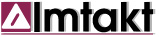IMTAKT HPLC TOOLS
 Sample Solution Calculator This is a tool for sample solution preparation. Fill out all relevant fields leaving the value you want to calculate blank. Next, choose the appropriate unit from the provided list, and then press “CALCULATE”. Enter the molar mass if you would also like to calculate the molar concentration. Molar Mass / Mol Wt:  g/mol      Solute:     µg mg (µL) g (mL)   Soln.Vol:     µL mL  L   Concentration:       Concentration Unit   pg/mL  ng/mL   µg/mL   mg/mL   µg/L (ppb)    mg/L (ppm)     g/L   pmol/mL nmol/mL µmol/mL mmol/mL   nM  µM   mM  M (mol/L)
 Copyright: I.YAZAWA, Imtakt Corp., JAPAN